Chin. Phys. B, 2020, Vol. 29(11): 110308    DOI: 10.1088/1674-1056/abbbde
 GENERAL Prev   Next

# Thermal entanglement in a spin-1/2 Ising–Heisenberg butterfly-shaped chain with impurities

Meng-Ru Ma(马梦如), Yi-Dan Zheng(郑一丹), Zhu Mao(毛竹), and Bin Zhou(周斌)
Department of Physics, Hubei University, Wuhan 430062, China
 Abstract  We investigate the effect of impurities on the thermal entanglement in a spin-1/2 Ising–Heisenberg butterfly-shaped chain, where four interstitial Heisenberg spins are localized on the vertices of a rectangular plaquette in a unit block. By using the transfer-matrix approach, we numerically calculate the partition function and the reduced density matrix of this model. The bipartite thermal entanglement between different Heisenberg spin pairs is quantified by the concurrence. We also discuss the fluctuations caused by the impurities through the uniform distribution and the Gaussian distribution. Considering the effects of the external magnetic field, temperature, Heisenberg and Ising interactions as well as the parameter of anisotropy on the thermal entanglement, our results show that comparing with the case of the clean model, in both the two-impurity model and the impurity fluctuation model the entanglement is more robust within a certain range of anisotropic parameters and the region of the magnetic field where the entanglement occurred is also larger. Keywords:  thermal entanglement      Ising-Heisenberg butterfly-shaped chain      impurities      transfer-matrix approach Received:  13 August 2020      Revised:  01 September 2020      Accepted manuscript online:  01 January 1900 Fund: the National Natural Science Foundation of China (Grant No. 12074101), the Science Fund for the New Century Excellent Talents in University of the Ministry of Education of China (Grant No. NCET-11-0960), and the Specialized Research Fund for the Doctoral Program of Higher Education of China (Grant No. 20134208110001). Corresponding Authors:  †Corresponding author. E-mail: maozhu@hubu.edu.cn ‡Corresponding author. E-mail: binzhou@hubu.edu.cn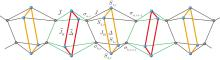Fig. 1.  A schematic representation of the spin-1/2 Ising–Heisenberg butterfly-shaped chain. The blue balls denote the Heisenberg particles and the red (orange) lines represent the Heisenberg interaction with (without) impurity, while the black balls denote the Ising spins and the green (black) lines represent the Ising interaction with (without) impurity.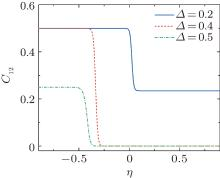Fig. 2.  Concurrence C12 as a function of the impurity parameter η for different values of Δ. Here, JH = 2J, J = 1, T = 0.1, B = 4, α = 0, and γ = 0.5.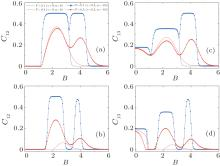Fig. 3.  Concurrences C12 and C13 as a function of the external magnetic field B for different values of temperature T. Here, JH = 2J, J = 1, and α = 0. In panels (a) and (c) we display concurrence for Δ = 0.4, panels (b) and (d) for Δ = 0.5. The solid lines and dot lines correspond to the clean model and the two-impurity model, respectively.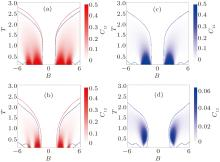Fig. 4.  Density plot of concurrence C12 as a function of the external magnetic field B and temperature T with JH = 2J and J = 1. In panels (a) and (b) we display C12 for the model with two impurities, where α = 0, γ = 0.5, and η = –0.5. In panels (c) and (d) we display C12 for the clean model, where α = 0, γ = 0, and η = 0. We set Δ = 0.4 in panels (a) and (c), Δ = 0.5 in panels (b) and (d), respectively. The red and blue solid curves are the contours of C12 = 0 which correspond to the two-impurity model and the clean model, respectively.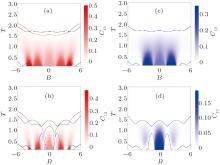Fig. 5.  Density plot of concurrence C13 as a function of the external magnetic field B and temperature T with JH = 2J and J = 1. In panels (a) and (b) we display C13 for the model with two impurities, where α = 0, γ = 0.5, and η = –0.5. In panels (c) and (d) we display C13 for the clean model, where α = 0, γ = 0, and η = 0. We set Δ = 0.4 in panels (a) and (c), Δ = 0.5 in panels (b) and (d), respectively. The red and blue solid curves are the contours of C13 = 0 which correspond to the two-impurity model and the clean model, respectively.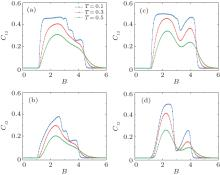Fig. 6.  The concurrence C12 as a function of the external magnetic field B under different values of temperature T with impurity fluctuations. Here, JH = 2J, J = 1 and α = 0. In panels (a) and (b) we present the uniform distribution for two impurities, and the Gaussian distribution in panels (c) and (d). For panels (a) and (c) Δ = 0.4, panels (b) and (d) Δ = 0.5. We take the average of 1000 calculations here.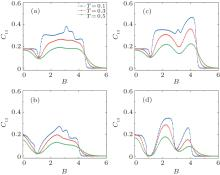Fig. 7.  The concurrence C13 as a function of the external magnetic field B under different values of temperature T with impurity fluctuations. Here, JH = 2J, J = 1 and α = 0. In panels (a) and (b) we present the uniform distribution for two impurities, and the Gaussian distribution in panels (c) and (d). For panels (a) and (c) Δ = 0.4, panels (b) and (d) Δ = 0.5. We take the average of 1000 calculations here.